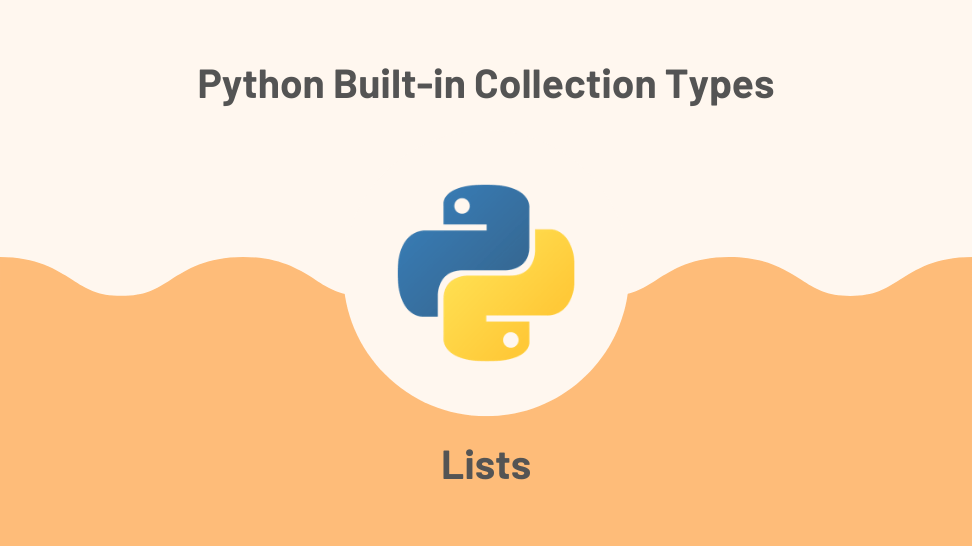In this article, I’ll explore lists, one of 4 built-in types used to store collections in Python.

This post is the first article in a miniseries exploring the topic of built-in collection types in Python. I based the series on my notes while studying for a Python technical interview.

For quick access, here is the list of posts on this series:

2. Python Tuples
3. Python Sets
4. Python Dictionaries

## Introduction to Lists

In Python, a list is a data type used for storing a collection of objects. Lists have the following characteristics:

### Lists have an order

Lists preserve the order of their elements. Two lists with the same elements but in different order are not equal:

### Lists can contain any arbitrary object

List elements can be of any data type. The example below shows a list containing integers, floats, boolean, strings, dictionaries, and other lists:

### Lists can contain duplicates

The same element can exist at different indexes in a list. For example, the list below contains the integer 1 at positions 0 and 2:

### Lists are mutable

After creating a list, you can change it at will. Lists come with many built-in methods that allow you to modify them. The example below uses the .append() method to add an element to the list:

### Lists are dynamic

Lists grow and shrink as needed when adding and removing elements:

## Creating a list

You can create a list using a list literal by wrapping its elements in square brackets:

You can also use the list() constructor:

## Accessing elements from a list

You can access an element from a list by using the element index. The index indicates the position of the element within the list. Indexes in sequential data types in Python start at 0.

## List slicing

By slicing a list, you can obtain a new list that is a sub-list of the original. The syntax for slicing is:

Which would return a sub-list from index = start to index = (end -1) using the given step (or 1 if no step is given).

Let’s look a some examples.

## List built-in methods

### list.append(x)

The .append(x) method adds an item to the end of the list. This method has a constant time complexity of O(1).

### list.extend(iterable)

The .extend(iterable) method appends all elements in the iterable to the original list. This method has a linear time complexity of O(k), where k is the length of the iterable.

### list.insert(i, x)

The .insert(i, x) method inserts item x in position i of the list. This method has a linear time complexity of O(n), where n is the length of the list.

### list.remove(x)

The .remove(x) method removes the first element with the value x from the list. It raises a ValueError if x is not in the list. This method has a linear time complexity of O(n), where n is the length of the list.

### list.pop(i)

The .pop(i) method returns the element in position i and removes it from the list. If you don’t pass i to the method, it removes the last element in the list. This method has a constant time complexity O(1) when popping from the end and a linear time complexity O(n) when popping from i, where n is the length of the list.

### list.clear()

The .clear() method removes all elements from the list. This method has a linear time complexity of O(n), where n is the length of the list.

### list.index(x)

The .index(x) method returns the index of the first occurrence of x in the list. This method has a linear time complexity of O(n), where n is the length of the list.

### list.count(x)

The .count(x) method returns the number of times x appears in the list. This method has a linear time complexity of O(n), where n is the length of the list.

### list.sort()

The .sort() method sorts the list in place. This method has a linear-logarithmic time complexity of O(n log n), where n is the length of the list.

### list.reverse()

The .reverse() method reverses the order of the list in place. This method has a linear time complexity of O(n), where n is the length of the list.

### list.copy()

The .copy() method returns a shallow copy of the list. This method has a linear time complexity of O(n), where n is the length of the list.

## List comprehensions

List comprehensions provide a concise way to create new lists by applying some operation or filter to another sequence or iterable. Let’s look at a few examples.

### Creating a list of squares

Say we need a list of the squares of the integers in another list. We could use an iterative solution such as:

Or we could use the following list comprehension, applying the x**2 operation to each element in the original list. The list comprehension is shorter, more readable, and more expressive than the iterative solution:

### Creating a list of even numbers

Below is an iterative solution for creating a list of even numbers from another list of numbers:

We can apply a filter to a list comprehension to achieve the same result in a concise way:

## Conclusion

In this article, we covered Python lists, a built-in data type you will most likely use in any non-trivial coding project. We covered the main characteristics of lists, the topic of list slicing, the list’s built-in methods with their time complexity, and briefly touched on list comprehension.